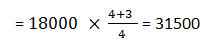## Sunday, 5 March 2017

### DI QUIZ12:BASED ON NEW PATTERN

Directions ( 1-5): Study the table carefully answer the questions given below.
Following table shows the percentage population of six states below poverty line and the proportion of males and females?

 Proportion of male and female State Percentage population below poverty line Below poverty line M: F Above poverty line M: F S₁ 16 4:3 3:2 S₂ 18 3:4 5:7 S₃ 26 2:3 4:5 S₄ 28 5:6 1:2 S₅ 12.5 3:2 6:5 S₆ 36 4:5 2:3

1. If the total population of state S₁ is 4400, then what is the approximate number of females above the poverty line in state S₁?
1. 1478
2. Other than the given options
3. 1578
4. 1484
5. 1487

2. If the total population of state S₃ and s₄ together is 17000, then what is the total number of females below the poverty line in the above-mentioned states?
1. 1320
2. 6820
3. 4850
5. Other than the given options

3. If the population of males below the poverty line in state S₁ is 18000 and that in state S₅  is 24000, then what is the ratio of the total population of state S₁ to that of state S₅?
1. 315:512
2. 316:513
3. Other than the given options
4. 315:513
5. 319:512

4. If the population of males above the poverty line in state S₂ is 4100 then what is the total population of that state?
1.Other than the given options
2. 12500
3. 13000
4. 14000
5. 12000

5. If in state S₆ the population of females above the poverty line in 4800 then what is the population of males below the poverty line in that state?
1. 2400
2. 2000
3. 2500
4. Other than the given options
5. 2800

1. 1;
Number of females above poverty line2. 4;
Since we cannot find the population of states separately, so we cannot find the required answer.

3. 1;
Population of state S₁ below poverty lineTotal population of state S₁Population of state S₅ below poverty linePopulation of state S₅∴ Required ratio = 196874: 320000 = 315: 512

4. 5;
Total population of state S₂5. 2;
Number of males below poverty line